#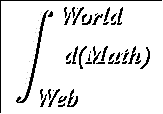The Squeeze Theorem

Suggested Prerequesites: Formal look at limits

Our immediate motivation for the squeeze theorem is to so that we can evaluate the following limits, which are necessary in determining the derivatives of sin and cosine:

#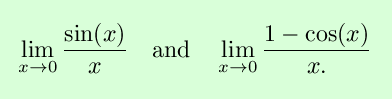The squeeze theorem is applied to these very useful limits on the page Useful Trig Limits.
The Squeeze Theorem:

If there exists a positive number p with the property that

#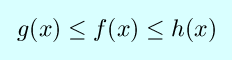for all x that satisfy the inequalities

then

#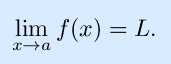Proof (nonrigorous):

This statement is sometimes called the ``squeeze theorem'' because it says that a function ``squeezed'' between two functions approaching the same limit L must also approach L.Intuitively, this means that the function f(x) gets squeezed between the other functions. Since g(x) and h(x) are equal at x = a, it must also be the case that f(x)=f(x)=L since there is no room for x to be anything else.

For the formal proof, let epsilon be given, and chose positive numbers

#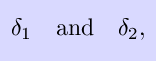both less than p, so that

Define

#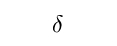to be the smallest of the numbers

Then

implies

#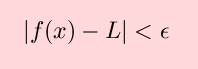and the proof is complete.

The Squeeze Theorem applied to Trig Limits | Back to the Calculus page
Back to the World Web Math top page

watko@mit.edu